Categories

## Compressing VGG for Style Transfer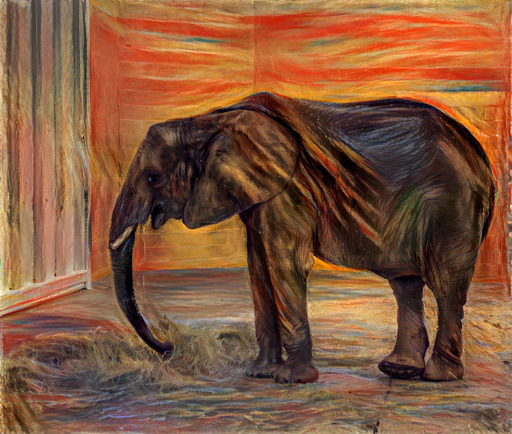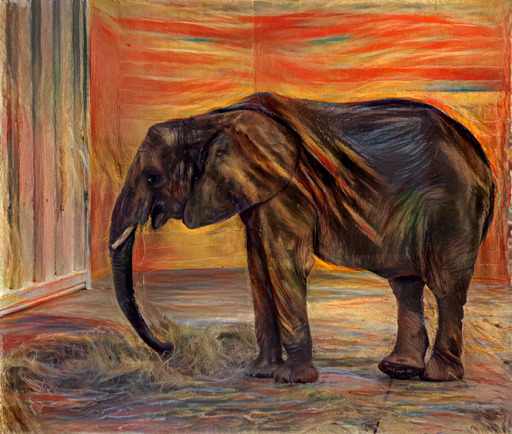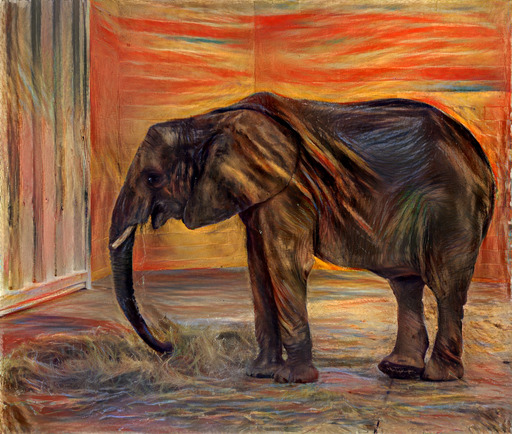32-bit float (no quantization) 8-bit 7-bit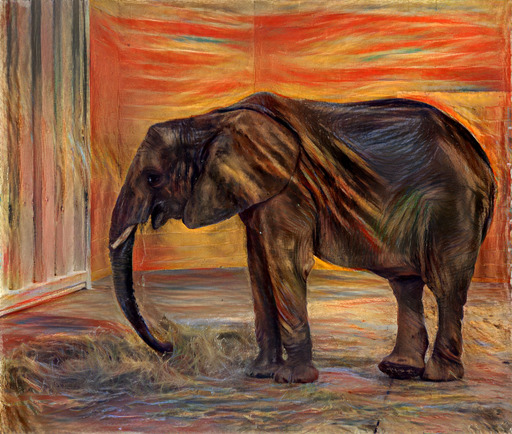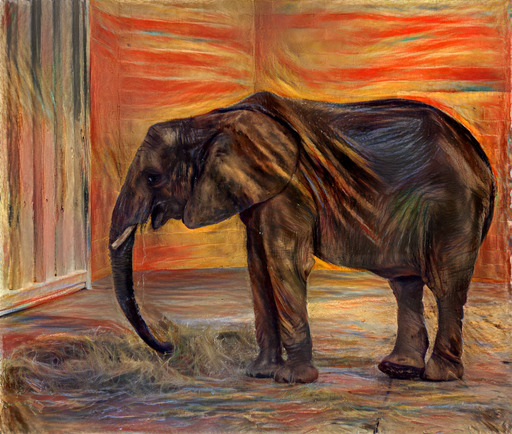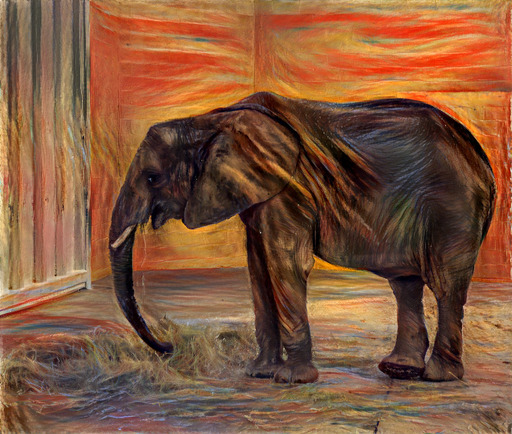6-bit 5-bit 4-bit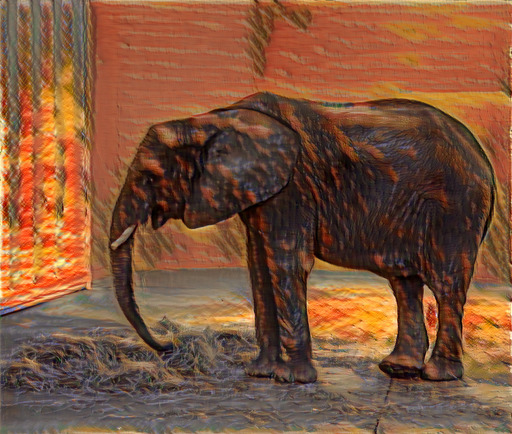3-bit 2-bit 1-bit

I recently implemented pastiche—discussed in a prior post—for applying neural style transfer. I encountered a size limit when uploading the library to PyPI, as a package cannot exceed 60MB. The 32-bit floating point weights for the underlying VGG model  were contained in an 80MB file. My package was subsequently approved for a size limit increase that could accommodate the VGG weights as-is, but I was still interested in compressing the model.

Various techniques have been proposed for compressing neural networks—including distillation  and quantization [3,4]—which have been shown to work well in the context of classification. My problem was in the context of style transfer, so I was not sure how model compression would impact the results.

#### Experiments

I decided to experiment with weight quantization, using a scheme where I could store the quantized weights on disk, and then uncompress the weights to full 32-bit floats at runtime. This quantization scheme would allow me to continue using my existing code after the model is loaded. I am not targeting environments where memory is a constraint, so I was not particularly interested in approaches that would also reduce the model footprint at runtime. I used kmeans1d—discussed in a prior post—for quantizing each layer’s weights.

Categories

## kmeans1d: Globally Optimal Efficient 1D k‑means Clustering

I implemented kmeans1d, a Python library for performing k-means clustering on 1D data, based on the algorithm from Xiaolin (1991), as presented by Grønlund et al. (2017, Section 2.2).

Globally optimal k-means clustering is NP-hard for multi-dimensional data. Lloyd’s algorithm is a popular approach for finding a locally optimal solution. For 1-dimensional data, there are polynomial time algorithms.

kmeans1d contains an O(kn + n log n) dynamic programming algorithm for finding the globally optimal k clusters for n 1D data points. The code is written in C++—for faster execution than a pure Python implementation—and wrapped in Python.

The source code is available on GitHub:
https://github.com/dstein64/kmeans1d

Categories

## k-means Image Color Quantization

I implemented a web page that can apply color quantization to images using k-means clustering. Here’s the link:
https://dstein64.github.io/k-means-quantization-js/

The JavaScript source code is available on GitHub:
https://github.com/dstein64/k-means-quantization-js

Categories

## Factorization Machines with Theano

Update 11/4/2019: The github repo was renamed from PyFactorizationMachines to pyfms.

Update 4/20/2017: The library is now available on PyPI, the Python Package Index. It can be installed with pip.

\$ pip install pyfms

A Factorization Machine (FM) is a predictive model that can be used for regression and classification (Rendle 2010). FMs efficiently incorporate pairwise interactions by using factorized parameters.

PyFactorizationMachines is a Theano-based Python implementation of factorization machines. documentation, see documentation.md.

For example usage, see example.py.

Categories

## Matrix Factorization with Theano

Matrix factorization algorithms factorize a matrix D into two matrices P and Q, such that D ≈ PQ. By limiting the dimensionality of P and Q, PQ provides a low-rank approximation of D. While singular value decomposition (SVD) can also be used for this same task, the matrix factorization algorithms considered in this post accommodate missing data in matrix D, unlike SVD.

For an overview of matrix factorization, I recommend Albert Au Yeung’s tutorial. That post describes matrix factorization, motivates the problem with a ratings prediction task, derives the gradients used by stochastic gradient descent, and implements the algorithm in Python.

For exploratory work, it would be great if the algorithm could be implemented in such a way that the gradients could be automatically derived. With such an approach, gradients would not have to be re-derived when e.g., a change is made to the loss function (either the error term and/or the regularization term). In general, automatically derived gradients for machine learning problems facilitate increased exploration of ideas by removing a time-consuming step.

Theano is a Python library that allows users to specify their problem symbolically using NumPy-based syntax. The expressions are compiled to run efficiently on actual data. Theano’s webpage provides documentation and a tutorial.

The following code includes a Theano-based implementation of matrix factorization using batch gradient descent. The parameters are similar to those in the quuxlabs matrix factorization implementation. D is a second-order masked numpy.ndarray (e.g., a ratings matrix, where the mask indicates missing ratings), and P and Q are the initial matrix factors. The elements of P and Q are the parameters of the model, which are initialized by the function’s caller. The rank of the factorization is specified by the dimensions of P and Q. For a rank-k factorization, P must be$m \times k$ and Q must be$k \times n$ (where D is an$m \times n$ matrix). Additional parameters specify the number of iterations, the learning rate, and the regularization importance.

The code doesn’t contain any derived gradients. It specifies the loss function and the parameters that the loss function will be minimized with respect to. Theano figures out the rest!

This file contains bidirectional Unicode text that may be interpreted or compiled differently than what appears below. To review, open the file in an editor that reveals hidden Unicode characters.